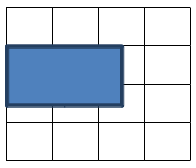### Area & perimeter activities - a teacher's experience with Math Mammoth

Ms. Pineda used two lessons from my book Mamut Matemáticas Geometría inicial with her bilingual class. Below is her lesson plan, and some reflections. I asked her some questions.

How did the children like the activities?

The worksheets were a great guide for them because they kept them focused, and the amount of , "What do I do?" questions were very minimal. They seemed comfortable referring back to the worksheets for definitions and examples. I also noticed there was a lot less hand-raising.

How did you like using Math Mammoth materials?

It definitely made my job easier, because I did not have to continue going back to each group to explain what are or perimeter were. The definition was in front of them along with examples.

How many class periods did you use them?
It took us two 45-minute periods to complete all the activities. The students were so engaged they did not want to quit.

Did you use both English and Spanish, or only Spanish worksheets?
I only used the Spanish versions, but I plan on assigning the English for homework when we return in January.

Did you give them any homework?
They were able to keep their booklets in their math folder in order to refer to them in the future. They will usually go back and complete any unfinished assignments.

LESSON PLANS & PICTURES

AREA

Area and Perimeter Activities
Ms. Pineda, 3rd Grade Bilingual Teacher

Objective: Measure the area of geometric shapes using square units.

Materials: Comenzando con área worksheet (a lesson from Mamut Matemáticas Geometría inicial),, attribute blocks, ¼ - ½ inch graphing paper, pencil, mini booklet made from manila paper, markers or crayons.

Procedures:
1. Use the worksheet to discuss how area is calculated.
2. Trace a shape using your pencil.
3. Count the whole squares by writing the numbers.
4. Combine two halves to make a whole square.
5. If one of your shapes crosses four squares, combine them to make a whole.
6.Area = 4 + ½ + ½ = 5

7. Once you have calculated the area of each shape. You may color it and glue it in your mini booklet.

PERIMETER

Objective: Measure the perimeter of geometric shapes in inches.

Materials: Perímetro worksheet (a lesson from Mamut Matemáticas Geometría inicial), attribute blocks, pencil, ruler, mini booklet made from manila paper, markers, and crayons.

Procedures:
1. Use the worksheet to discuss how perimeter is calculated.
3. Measure each side of the shape in inches.
4. Add the lengths to find the perimeter of each shape.
5. Combine two halves (½ + ½ = 1)to make a whole inch.
6. Trace the edges of each shape with a marker and color the area with a crayon.
Perímetro = 2 + 2 + 1 + 1 + ½ + ½ = 7